StatLect

# Sample mean

The sample mean is a statistic obtained by calculating the arithmetic average of the values of a variable in a sample.

If the sample is drawn from probability distributions having a common expected value, then the sample mean is an estimator of that expected value.## Definition

A more precise definition follows.

Definition Letbe the observed values of a variable. Their sample mean, denoted by, is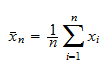The sample mean is a fundamental quantity in statistics. Its properties are discussed in the next sections.

## The sampling distribution

In order to analyze the properties of the sample mean, we assume thatare the realizations ofrandom variables.

We use the term sample mean also when we refer to the random variable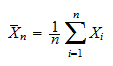In other words, before the realizations of the random variables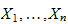become known, their sample mean can be regarded as a random variable.

The probability distribution ofis called the sampling distribution of the sample mean.

## Population mean

Ifall have the same expected value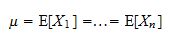thenis called the population mean.

## The sample mean as an estimator

When the population meanis unknown, the realizationis an estimate of, while the random variableis an estimator of(remember that an estimator is a pre-defined rule that associates an estimate to each possible sample we can observe).

## Expected value

The first important property of the sample mean is that it is an unbiased estimator of the population mean: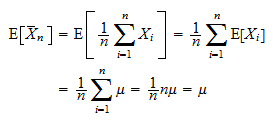## Variance

Suppose that the random variablesare independent and have a common finite variance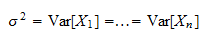Then, the variance of the sample mean is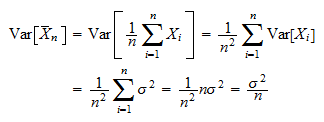## Law of large numbers

Under appropriate conditions, the sample mean converges (in probability or almost surely) to the population mean.

This fundamental result is known as Law of Large Numbers.

## Consistent estimator

When the estimator of a parameter converges to the true value of the parameter, we say that it is consistent.

Therefore, if a law of large numbers applies, the sample mean is a consistent estimator of the population mean.

## Central limit theorem

Under appropriate conditions, the random variable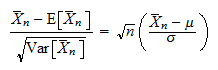converges in distribution to a standard normal distribution (i.e., a normal distribution with zero mean and unit variance).

This is another fundamental result, known as Central Limit Theorem.

## Asymptotically normal estimator

When a central limit theorem applies, and the statistic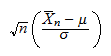converges to a normal distribution, we say that the sample mean is asymptotically normal.

## Exact distribution

If the random variablesare independent normal random variables, then also the sample mean has a normal distribution.

This is discussed in the lecture on mean estimation.

## Maximum likelihood estimator

In many important cases, the sample mean coincides with the maximum likelihood estimator (MLE) of the population mean. See, for example:

## More details

Want to know more about the sample mean? See how it is used to:

Previous entry: Robust standard errors

Next entry: Sample point